### THE DESIGN PROTOCOL: PART 3j,CROSSOVERS - SPEAKER IMPEDANCE EQUALIZATION

The last part of our design protocol deals with impedance correction or compensation that many engineers also call impedance equalization. It addresses the major problem of speaker impedance fluctuation with frequency. Generally speaking speaker impedance is not a fixed value independent of frequency as we would like it to be.

We have already discussed the way the impedance of a single loudspeaker changes with frequency. It incorporates a peak due to suspension resonance and a rise at high frequencies due to voice coil inductance. Woofers have their resonance peaks at low frequencies below 100-200Hz while tweeters exhibit resonance in a wide frequency range above 500-600Hz. These impedance peaks interfere with crossover filter operation only at frequencies where the latter introduces signal roll-off. A detailed investigation of this issue was given back at Part 3c.

-When crossover design is finished how does the speaker's impedance curve look like ?

The following picture gives us the impedance plot for typical speaker system when crossover filters are finished. As in most speaker cases a peak is formed above 1kHz. In this case it is located at 1500Hz. In most cases two relatively flat impedance 'plateaus' are formed at both sides around this peak.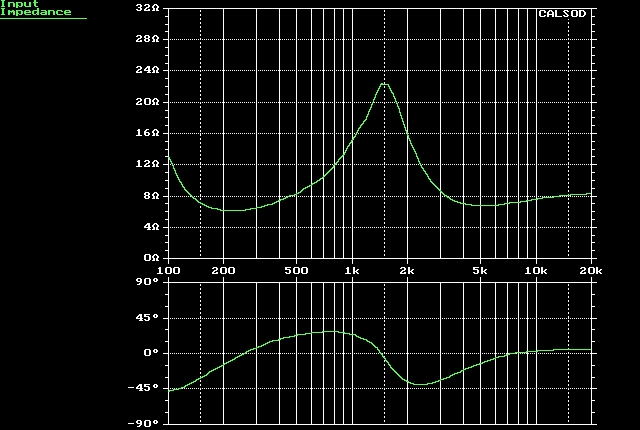-Is this peak due to tweeter impedance resonance ? If not what is its origin ?

No it is not. What actually happens in all crossover networks in terms of electric current flow is that as frequency rises the low-pass filter suppresses electric current and the high-pass filter starts conducting it. In the crossover frequency range these two effects work in a complementary way so that total current flow is generally preserved.

However in most crossover circuits there are always narrow frequency regions where the filters fail to complement their current flow changes. In such frequencies overall current flow decreases (total impedance rise) or increases (total impedance fall). That is why peaks or dips are formed in the speaker's total impedance response curve.

Depending on the crossover circuit topology we may also have an additional 'inductive' rise of impedance above 4-5kHz. It can also be corrected through an additional RC subcircuit placed also in parallel to our crossover filters. The design of such a circuit has been discussed in Part 3c and will be omitted.

-Do we always have some sort of a peak in speaker impedance curves ?

No. We could have a dip down to unacceptable impedance values : 2-4 Ohms for an '8 Ohm' speaker. In such a case a redesign of crossover filters should be attempted with shifting away their cut-off frequencies.

-So how does an 'Impedance Correction Scheme' (TM) actually work ?

It is a simple idea where another subcircuit is placed in parallel to our finished crossover filter circuits. This circuit presents its own impedance and draws its own current. What we usually do is to make this circuit draw current in the narrow frequency region where our crossover network exhibits an impedance peak. Outside this region we make this circuit draw very little or no current at all.

In this way the 'combined' impedance decreases in the frequency range where the peak was originally present. The 'combined' impedance curve becomes as flat as possible (compensated).

-What sort of subcircuit is suitable for the equalization of impedance peaks ?

There can not be a straightforward answer unless we find the mathematical 'model' of an impedance curve. It is a very good approximation to assume that this curve is actually formed by an ohmic 'substrate' (fixed resistance R1) over which a resonant parallel RLC 'adds' its peak. This electrical model is presented below: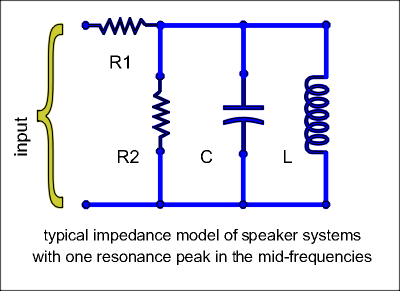Its impedance plot (given below) exhibits a flat and fixed value (equal to R1) at low and high frequencies far away from resonance frequency fo. At resonance, impedance climbs to a peak value of R1+R2. When logarithmic frequency axis is used impedance curve is almost symmetrical around fo.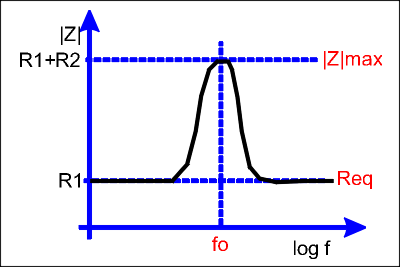In real world speakers impedance curves can be approximated by this model inside a relatively wide frequency range to a good degree of accuracy. In that frequency range an equalization subcircuit exists : a series RLC circuit. The figure that follows illustrates such a subcircuit: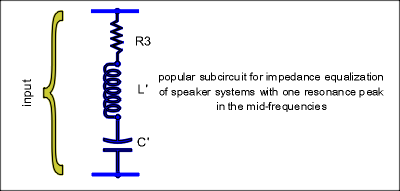-How do we evaluate the component values of this series RLC circuit during real-world speaker design ?

We need the curve of the overall speaker impedance with its crossover network finalized. It can be either simulated by our design software or measured as soon as the crossover pcb is assembled. In this curve we will notice a peak impedance value and flat impedance plateaus at both sides around the resonance peak. These flat areas do not necessarily occur at the same impedance value in Ohms because they reflect different drivers and filters. The left one is associated with the woofer (and its LPF) while the right one is associated to the tweeter and its HPF.

Since in our model there is only one resistance value for both plateaus (decided by R1) and it is this value at which the equalization will fix the speaker's impedance, we usually select the highest of these two values. We call it Req and in some cases we even set it equal to 7-8 Ohms arbitrarily.

Then we mark an impedance value |Z*| at a frequency f* higher than fo. As a rule we choose impedance values somewhere in the middle between R1 and |Z|max.

We will use the following figure as a reference guide:We may need to zoom in a narrow frequency range around the resonance frequency. In this way we will be able to get a good estimate of fo, |Z|max, f* and |Z*|.

Then we use the set of formulas given below: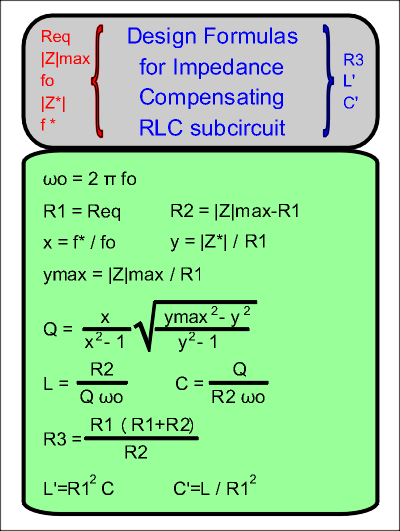Q expresses the quality factor of the resonance peak that needs to be equalized. L and C are those described in our theoretical model. Finally we get R3, L' and C' component values for the equalization RLC subcircuit.

Needless to say that R3, L' and C' values should be truncated to the nearest stock values available in the market. They should also be tested in our simulation software to make sure they do not affect (ie. to lower) the nearby impedance plateaus in a bad sense. After all our theoretical model could easily fail to describe the peak in our speaker impedance curve. In such a case the RLC equalization subcircuit may well be a failure too.

Under all circumstances we can use our simulation software to automatically optimize our RLC subcircuit in the mid-frequencies.

www.diy-loudspeakers.com

### Who is online

We have 48 guests and no members online

### Visitors since 8/4/1541.0% USA4.0% Germany3.9% United Kingdom3.5% China3.1% Russia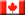3.1% Canada3.0% France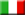3.0% Italy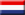2.8% Netherlands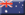2.6% Australia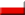2.3% Poland1.6% Sweden

Total:

202

Countries
256207
Today: 3
Yesterday: 114
This Month: 2,524
Last Month: 3,752